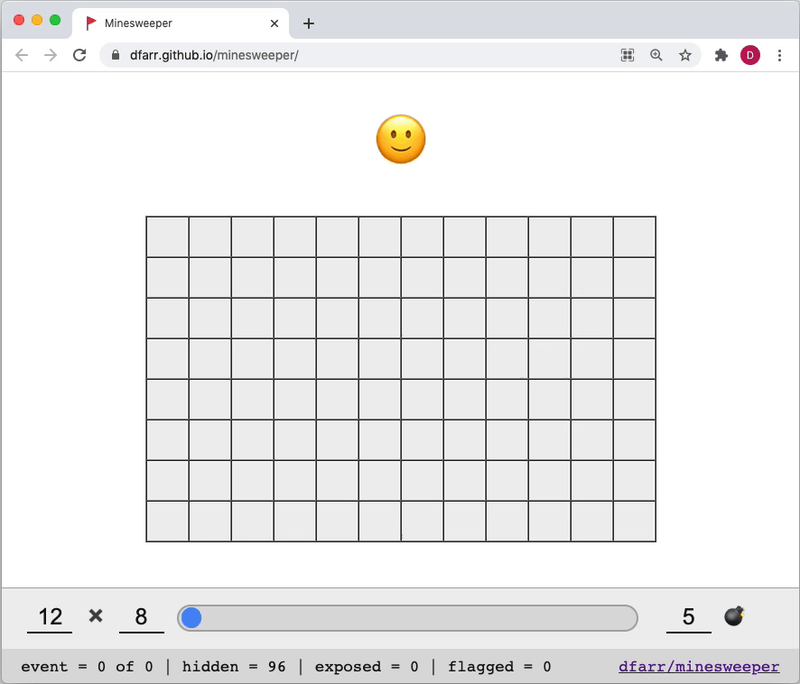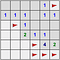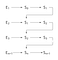# Event Sourcing Minesweeperdfarr.github.io/minesweeper

At some point during the stay at home order in California last year I found myself with enough free time to try something I’ve always to do, implement my own version of the classic game Minesweeper. This is the story of creating an event sourced Minesweeper written in Elm.

# Event Sourcing

Capture all changes to an application state as a sequence of events.

In Martin Fowler’s seminal post on the matter, he declares that event sourcing applications save all changes to application state as a sequence of events. These events can later be queried to answer such questions as “how did we get here?”.

Since this is purely a fun project, this post will focus less on technology and more on functional implementation. I have chosen Elm for this project, a purely functional language that compiles to javascript. There will be no web server, we will store the sequence of events in memory and all computation will occur in the browser.

# VisionHere we can deduce all flagged squares must contain a mine using the information provided by the revealed squares.

In our version of Minesweeper we will implement the game in it’s classical form, but with a twist. Our version will include a slider that the player can drag to reverse the game to any previous state. We will achieve our vision by replaying the event stream from an initial state to the desired state.

# Initial Implementation

First, let’s define our state.

`type alias Grid a  = List (List a)type State  = Initial  | Pending (Grid Square)  | Success (Grid Square)  | Failure (Grid Square)type Square  = Hidden  Value  | Marked  Value  | Exposed Valuetype Value  = Safe Int  | Mine`

Next, let’s define our events.

`type alias Coord  = ( Int, Int )type Event  = Clicked Coord Square  | Flagged Coord Square`

Initially, all squares will be hidden to the player. When a player clicks or right clicks on a hidden square we will construct the corresponding event, passing the coordinates (a tuple of integers) as well as the square itself to the data constructor.

# Apply FunctionTo determine the next state Sn+1 we can apply the event En+1 to the previous state Sn. To determine any previous state we can fold the event stream from an initial state S₀ and a set of events {E₀, E₁, …, En}.

Let’s assume the logic to generate a `Grid Square` required to play our game is already defined. Let’s also assume this logic ensures the state is `Pending` once this initial setup is complete.

We need to handle the following scenarios:

• Player flags a square
• Player clicks on a square that is a mine
• Player clicks on a square that is not a mine
• Player clicks on a square that is not a mine and has no adjacent mines

These scenarios are listed in increasing specificity. We need to handle the final case in order to ensure that when a player clicks on a square that has no adjacent mines, the neighbouring squares are automatically clicked as well (as we know these cannot contain a mine). Let’s write some scaffolding.

`apply : Event -> State -> Stateapply event state = case ( state, event ) of  ( Pending _, Clicked _ (Hidden (Safe 0)) ) -> state  ( Pending _, Clicked _ (Hidden Mine) )     -> state  ( Pending _, Clicked _ (Hidden _) )        -> state  ( Pending _, Flagged _ (Hidden _) )        -> state  ( Pending _, Flagged _ (Marked _) )        -> state  _                                          -> state`

Notice that unlike above we need to list our cases in decreasing order of specificity to ensure that the more generic case is not matched before the specific case. Also notice that our game can only be played if the grid is in the `Pending` state.

Let’s take a slight detour to model the relationship between squares in a grid. In Minesweeper two squares may be neighbours or not neighbours. Additionally two squares may in fact be the same square. Let’s model this and write a function that returns the type of relationship between two sets of coordinates.

`type Relationship = Identity | Neighbor | Neitherrelationship : Coord -> Coord -> Relationshiprelationship ( i1, j1 ) ( i2, j2 ) =  case ( i1 - i2 |> abs, j1 - j2 |> abs ) of    ( 0, 0 ) -> Identity    ( 0, 1 ) -> Neighbor    ( 1, 0 ) -> Neighbor    ( 1, 1 ) -> Neighbor    _        -> Neither`The tuple shown within the square is the result of (i-2, j-2).

Here you can see the relationship between squares in a hypothetical grid that would occur if an event is triggered on the square with coordinates of `(2, 2)`. The blue square is the identity square, the yellow squares are the neighbouring squares, and grey squares are neither.

With this in place let’s write a function that maps a `Grid` given a set of coordinates and the following three functions as parameters:

• a function that maps the identity square
• a function that maps the neighbouring squares
• a function that maps all other squares
`type alias F a b  = ( Coord, a ) -> bindexedMap : F a b -> F a b -> F a b -> Coord -> Grid a -> Grid bindexedMap f1 f2 f3 c1 =  (\i j -> _indexedMap f1 f2 f3 c1 ( i, j ))    >> List.indexedMap    |> List.indexedMap_indexedMap : F a b -> F a b -> F a b -> Coord -> Coord -> a -> b_indexedMap f1 f2 f3 c1 c2 =  case relationship c1 c2 of    Identity -> f1 << Tuple.pair c2    Neighbor -> f2 << Tuple.pair c2    Neither  -> f3 << Tuple.pair c2`

Here, we are mapping over a `Grid Square` which is just a list of lists. We can use Elms standard `List.indexedMap` to extract the index of each `Square` as we go, if we do this twice we can combine both indices to construct a coordinate `(i, j)` . With this we have all information necessary to apply the correct mapping function. Notice we are combining the coordinates and the square itself into a tuple to pass to the mapping functions, this will come in handy later. Let’s define one more function for the special case when we only want to map the identity square to a known value.

`mapIdentity : a -> Coord -> Grid a -> Grid amapIdentity x =  indexedMap (always x) Tuple.second Tuple.second`

This function will map the identity square to a provided value `x` . We can use Elms standard `Tuple.second` function to return the `a` value of the tuple for all neighbouring and neither squares. Finally with all the above pieces, and the following state machine for reference, we can write our apply function!

`apply : Event -> State -> Stateapply event state =  case ( state, event ) of    ( Pending grid, Clicked coord (Hidden (Safe 0)) ) ->      grid        |> indexedMap (always Nothing) click (always Nothing) coord        |> List.concat        |> List.filterMap identity        |> List.foldl apply            (grid |> mapIdentity (Safe 0 |> Exposed) coord |> check)    ( Pending grid, Clicked coord (Hidden Mine) ) ->      grid        |> mapIdentity (Exposed Mine) coord        |> Failure    ( Pending grid, Clicked coord (Hidden value) ) ->      grid        |> mapIdentity (Exposed value) coord        |> check    ( Pending grid, Flagged coord (Hidden value) ) ->      grid        |> mapIdentity (Marked value) coord        |> Pending    ( Pending grid, Flagged coord (Marked value) ) ->      grid        |> mapIdentity (Hidden value) coord        |> Pending    _ -> stateclick : ( Coord, Square ) -> Maybe Eventclick ( coord, square ) =  case square of    Hidden _ -> Just (Clicked coord square)    _        -> Nothingcheck : Grid Square -> Statecheck grid =  case grid |> List.concat |> List.all found of    True  -> Success grid    False -> Pending gridfound : Square -> Boolfound square =  case square of    Exposed (Safe _) -> True    Hidden Mine      -> True    Marked Mine      -> True    _                -> False`

In every case we use the `mapIdentity` function to map the identity square to a new value. When a hidden square is clicked that is not a mine, we need to loop through all squares and determine if the game has been won and set the overall state accordingly.

When the player clicks on a square with no adjacent mines, we need to simulate a click for all hidden neighbouring squares. To achieve this we can use the more generic `indexedMap` to map all squares to a corresponding `Maybe Event` where non-neighbouring squares are mapped to `Nothing` and then filtered out. With this list of events in hand we can recursively call the apply function to fold each event (more on folds below) onto the current state, effectively clicking all neighbouring squares at once.

# Replaying The Event Stream

`type alias Model =  { state  : { initial : State, current : State }  , events : List Event  }`

Whenever an event occurs we execute the apply function, update the current state, and append the event to a list of all previous events. To replay the event stream to any past state we can use a left fold.

`replay : Model -> Int -> Statereplay { state, events } index =  events    |> List.take index    |> List.foldl apply state.initial`

The standard `List.foldl` function takes our apply function as a parameter and applies each event from our list of events to the output of the application of the previous event, starting with the initial state. Therefore, every time you move the slider one position all the events up to that point are re-applied in order from beginning to end in the exact same manner as the game was originally played!

# Thoughts

Check out the full source code at github.com/dfarr/minesweeper.